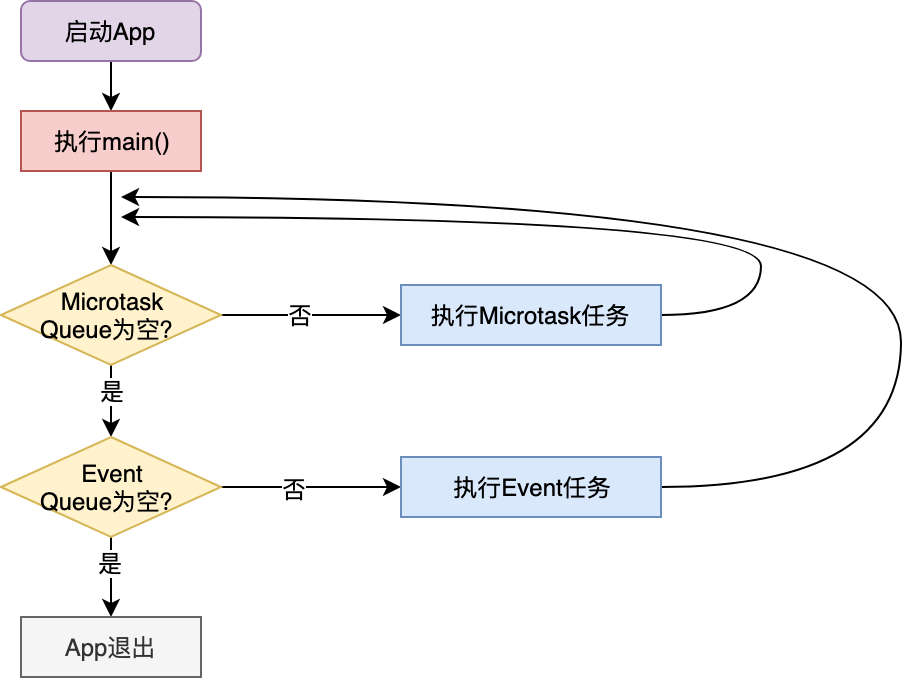Dart 是单线程的。

• Event Queue 事件队列，Flutter 为 Event Queue 的任务建立提供了一个封装，就是 Future

# Future

Future 对象表示异步操作结果，通常通过 then() 来处理返回的结果

## 结论

1. then 与 Future 函数体共用一个事件循环。如果 Future 有多个 then ，它们也会按照链式调用的先后顺序同步执行，同样也会共用一个事件循环。
2. 如果 Future 执行体已经执行完毕了，但你又拿着这个 Future 的引用，往里面加了一个 then ， Dart 会将后续加入的 then 方法体放入微任务队列，尽快执行。
3. 如果 then 中也是一个 Future ，那么这个 then ，以及后续的 then 都被放入到事件队列中了，
4. then 会在 Future 函数体执行完毕后立刻执行，无论是共用同一个事件循环还是进入下一个微任务。

## 例一

//声明了一个匿名 Future ，并注册了两个 then 。第一个 then 是一个Future
Future(() => print('f6'))
.then((_) => Future(() => print('f7')))
.then((_) => print('f8'));

//声明了一个匿名Future
Future(() => print('f9'));


f6   f9   f7   f8


## 例二

//f1比 f2 先执行
Future(() => print('f1'));
Future(() => print('f2'));

//f3执行后会立刻同步执行then 3
Future(() => print('f3')).then((_) => print('then 3'));

//then 4会加入微任务队列，尽快执行
Future(() => null).then((_) => print('then 4'));


main thread
f1
f2
f3
then 3
then 4



## 案例

Future(() => print('f1'));//声明一个匿名Future
Future fx = Future(() =>  null);//声明 Future fx ，其执行体为null

//声明一个匿名 Future ，并注册了两个 then 。在第一个 then 回调里启动了一个微任务
Future(() => print('f2')).then((_) {
print('f3');
}).then((_) => print('f5'));

//声明了一个匿名 Future ，并注册了两个 then 。第一个 then 是一个Future
Future(() => print('f6'))
.then((_) => Future(() => print('f7')))
.then((_) => print('f8'));

//声明了一个匿名Future
Future(() => print('f9'));

//往执行体为 null 的 fx 注册了了一个then
fx.then((_) => print('f10'));

//启动一个微任务
print('f12');


f12 f11 f1 f10   f2 f3 f5  f4  f6 f9  f7  f8

• 第一轮循环，只输出了 f12 ，其他的要么添加到事件队列中，要么添加到微任务队列中
• 第二轮循环，输出 f11 ，因为他在微任务队列中
• 第三轮循环，输出 f1 ， fx 执行，然后
• 第四次循环，输出 f10 ，因为 f10 的引用 fx 在上一轮执行了，那么再用这个 Future 引用，会把他们添加到微任务队列中，首先执行，所以会 输出 f10
• 第五轮循环， 输出 f2 f3 f5 ， f4 会添加到微任务队列中，
• 第六轮循环，输出 f4
• 第七轮循环，输出 f6 ， f9 ，同时 f7 添加到事件队列中
• 第八轮循环，输出 f7 f8

# 异步函数

Dart 中的 await 并不是阻塞等待，而是异步等待 。 Dart 会把调用体的函数也当做异步函数，将等待异步任务的上下文也放入到 Event Queue 中，一旦有了结果， Event Loop 就会把它从 Event Queue 中取出，等待代码继续执行。

## 例一

//声明了一个延迟 2 秒返回 Hello 的 Future ，并注册了一个 then 返回拼接后的Hello 2019
Future<String> fetchContent() =>
Future<String>.delayed(Duration(seconds:2), () => "Hello")
.then((x) => "\$x 2019");
//异步函数会同步等待 Hello 2019 的返回，并打印
func() async => print(await fetchContent());

main() {
print("func before");
func();
print("func after");
}


func before
func after
Hello 2019


func before
Hello 2019
func after


main() async{
print("func before");
print(await fetchContent());//等待 Hello 2019 的返回
print("func after");
}


## 例二

Future(() => print('f1'))
.then((_) async => await Future(() => print('f2')))
.then((_) => print('f3'));
Future(() => print('f4'));


f1  f4   f2   f3


main() async{
await Future(() => print('f1'))
.then((_)  =>  Future(() => print('f2')))
.then((_) => print('f3'));
Future(() => print('f4'));
}